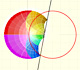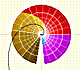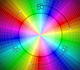Inversion in a circle is a transformation that preserves the magnitude of angles but each angle is mapped to an angle of opposite sign. The sense or direction of each angle is reversed. Inversion in a circle is an anticonformal mapping (like a reflection in a line).

In particular, inversion is a mapping that preserves the angle of intersection of two circles. Orthogonal circles (or lines) invert into orthogonal circles (or lines). A circle orthogonal to the circle of inversion is mapped into itself.All the lines through a point and all the circles concentric with the same point as a center can be seen as two particular cases of coaxal sistem of circles. These two coaxal sistems are orthogonal. The lines form a coaxal system of intersecting type and the concentric circles are a non-intersecting coaxal system. These coaxal systems are mapped into two orthogonal coaxal systems: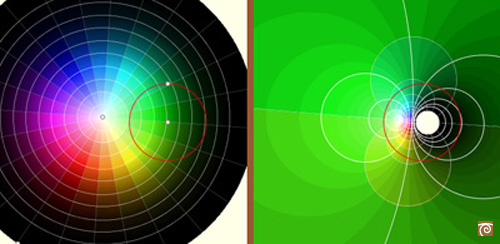Every "small" rectangle is transformed into an "small" rectangle:In this picture we can see how the order of colors is reversed: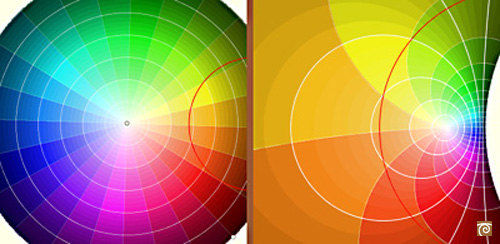REFERENCES

Hilber and Cohn-Vossen - Geometry and the Imagination (pag. 253) - Chelsea Publishing Company
Coxeter - Introduction to Geometry - Wiley and Sons.
Pedoe - Circles, a Mathematical View - Dover
Tristan Needham - Visual Complex Analysis. (pag. 124) - Oxford University Press
Rademacher and Toeplitz - The Enjoyment of Mathematics
Ogilvy - Excursions in Geometry (pag. 24)- Oxford University Press